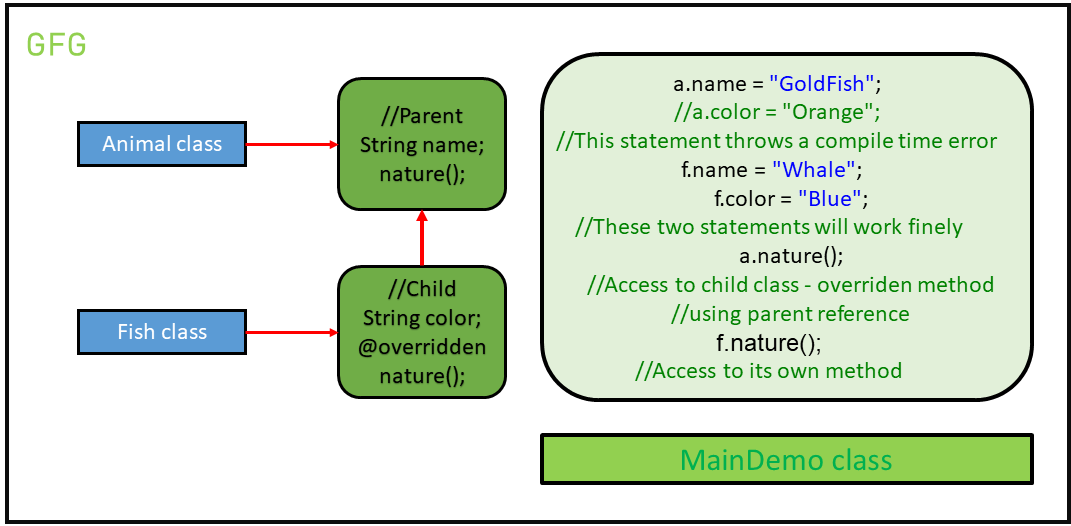Open in App
Not now

# Upcasting in Java with Examples

• Difficulty Level : Basic
• Last Updated : 29 Nov, 2022

Inheritance is an important pillar of OOP(Object Oriented Programming). It is the mechanism in Java by which one class is allowed to inherit the features (fields and methods) of another class. There are two ways in which the objects can be initialized while inheriting the properties of the parent and child classes. They are:

1. Child c = new Child(): The use of this initialization is to access all the members present in both parent and child classes, as we are inheriting the properties.
2. Parent p = new Child(): This type of initialization is used to access only the members present in the parent class and the methods which are overridden in the child class. This is because the parent class is upcasted to the child class.

What is upcasting? Upcasting is the typecasting of a child object to a parent object. Upcasting can be done implicitly. Upcasting gives us the flexibility to access the parent class members but it is not possible to access all the child class members using this feature. Instead of all the members, we can access some specified members of the child class. For instance, we can access the overridden methods. Example: Let there be an animal class. There can be many different classes of animals. One such class is Fish. So, let’s assume that the fish class extends the Animal class. Therefore, the two ways of inheritance, in this case, is implemented as: Let’s understand the following code to find out the difference:

## Java

 `// Java program to demonstrate``// the concept of upcasting` `// Animal Class``class` `Animal {` `    ``String name;` `    ``// A method to print the``    ``// nature of the class``    ``void` `nature()``    ``{``        ``System.out.println("Animal");``    ``}``}` `// A Fish class which extends the``// animal class``class` `Fish ``extends` `Animal {` `    ``String color;` `    ``// Overriding the method to``    ``// print the nature of the class``    ``@Override``    ``void` `nature()``    ``{``        ``System.out.println("Aquatic Animal");``    ``}``}` `// Demo class to understand``// the concept of upcasting``public` `class` `GFG {` `    ``// Driver code``    ``public` `static` `void` `main(String[] args)``    ``{``        ``// Creating an object to represent``        ``// Parent p = new Child();``        ``Animal a = ``new` `Fish();` `        ``// The object 'a' has access to``        ``// only the parent's properties.``        ``// That is, the colour property``        ``// cannot be accessed from 'a'``        ``a.name = "GoldFish";` `        ``// This statement throws``        ``// a compile-time error``        ``// a.color = "Orange";` `        ``// Creating an object to represent``        ``// Child c = new Child();``        ``Fish f = ``new` `Fish();` `        ``// The object 'f' has access to``        ``// all the parent's properties``        ``// along with the child's properties.``        ``// That is, the colour property can``        ``// also be accessed from 'f'``        ``f.name = "Whale";``        ``f.color = "Blue";` `        ``// Printing the 'a' properties``        ``System.out.println("Object a");``        ``System.out.println("Name: " + a.name);` `        ``// This statement will not work``        ``// System.out.println("Fish1 Color" +a.color);` `        ``// Access to child class - overridden method``        ``// using parent reference``        ``a.nature();` `        ``// Printing the 'f' properties``        ``System.out.println("Object f");``        ``System.out.println("Name: " + f.name);``        ``System.out.println("Color: " + f.color);``        ``f.nature();``    ``}``}`

Output:

```Object a
Name: GoldFish
Aquatic Animal
Object f
Name: Whale
Color: Blue
Aquatic Animal```

An illustrative figure of the program:• From the above example, it can be clearly understood that we can not access child class members using a parent class reference even though it is of the child type. That is:
```// This statement throws
// a compile-time error
a.color = "Orange";```
• And from the above example, we can also observe that we are able to access the parent class members and child class’s overridden methods using the same parent class reference object. That is:
```// Access to child class
// overridden method
a.nature();```
• Therefore, we can conclude that the main purpose of using these two different syntaxes is to get variation in accessing the respective members in classes.

My Personal Notes arrow_drop_up